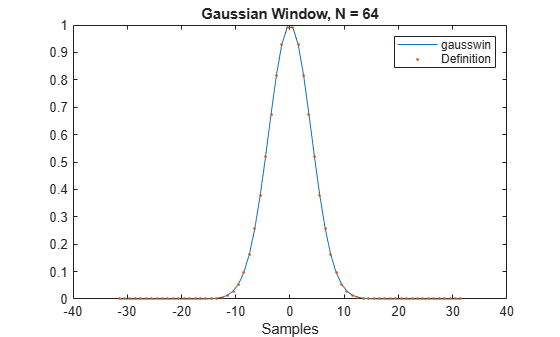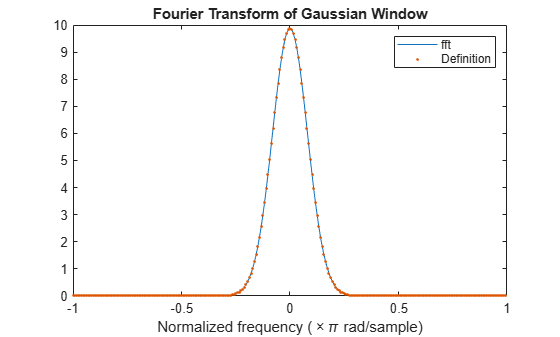gausswin

Gaussian window

Description

example

w = gausswin(L) returns an L-point Gaussian window.

w = gausswin(L,alpha) returns an L-point Gaussian window with width factor alpha.

Note

If the window appears to be clipped, increase L, the number of points.

Examples

collapse all

Create a 64-point Gaussian window. Display the result in wvtool.

L = 64;
wvtool(gausswin(L))This example shows that the Fourier transform of the Gaussian window is also Gaussian with a reciprocal standard deviation. This is an illustration of the time-frequency uncertainty principle.

Create a Gaussian window of length 64 by using gausswin and the defining equation. Set $\alpha =8$, which results in a standard deviation of 64/16 = 4. Accordingly, you expect that the Gaussian is essentially limited to the mean plus or minus 3 standard deviations, or an approximate support of [-12, 12].

N = 64;
n = -(N-1)/2:(N-1)/2;
alpha = 8;

w = gausswin(N,alpha);

stdev = (N-1)/(2*alpha);
y = exp(-1/2*(n/stdev).^2);

plot(n,w)
hold on
plot(n,y,'.')
hold off

xlabel('Samples')
title('Gaussian Window, N = 64')Obtain the Fourier transform of the Gaussian window at 256 points. Use fftshift to center the Fourier transform at zero frequency (DC).

nfft = 4*N;
freq = -pi:2*pi/nfft:pi-pi/nfft;

wdft = fftshift(fft(w,nfft));

The Fourier transform of the Gaussian window is also Gaussian with a standard deviation that is the reciprocal of the time-domain standard deviation. Include the Gaussian normalization factor in your computation.

ydft = exp(-1/2*(freq/(1/stdev)).^2)*(stdev*sqrt(2*pi));

plot(freq/pi,abs(wdft))
hold on
plot(freq/pi,abs(ydft),'.')
hold off

title('Fourier Transform of Gaussian Window')Input Arguments

collapse all

Window length, specified as a positive integer.

Data Types: single | double

Width factor, specified as a positive real scalar. alpha is inversely proportional to the width of the window.

Data Types: single | double

Output Arguments

collapse all

Gaussian window, returned as a column vector.

Algorithms

The coefficients of a Gaussian window are computed from the following equation:

$w\left(n\right)={e}^{-\frac{1}{2}{\left(\alpha \frac{n}{\left(L-1\right)/2}\right)}^{2}}={e}^{-{n}^{2}/2{\sigma }^{2}},$

where –(L – 1)/2 ≤ n ≤ (L – 1)/2, and α is inversely proportional to the standard deviation, σ, of a Gaussian random variable. The exact correspondence with the standard deviation of a Gaussian probability density function is σ = (L – 1)/(2α).

 Hansen, Eric W. Fourier Transforms: Principles and Applications. New York: John Wiley & Sons, 2014.

 Oppenheim, Alan V., Ronald W. Schafer, and John R. Buck. Discrete-Time Signal Processing. Upper Saddle River, NJ: Prentice Hall, 1999.